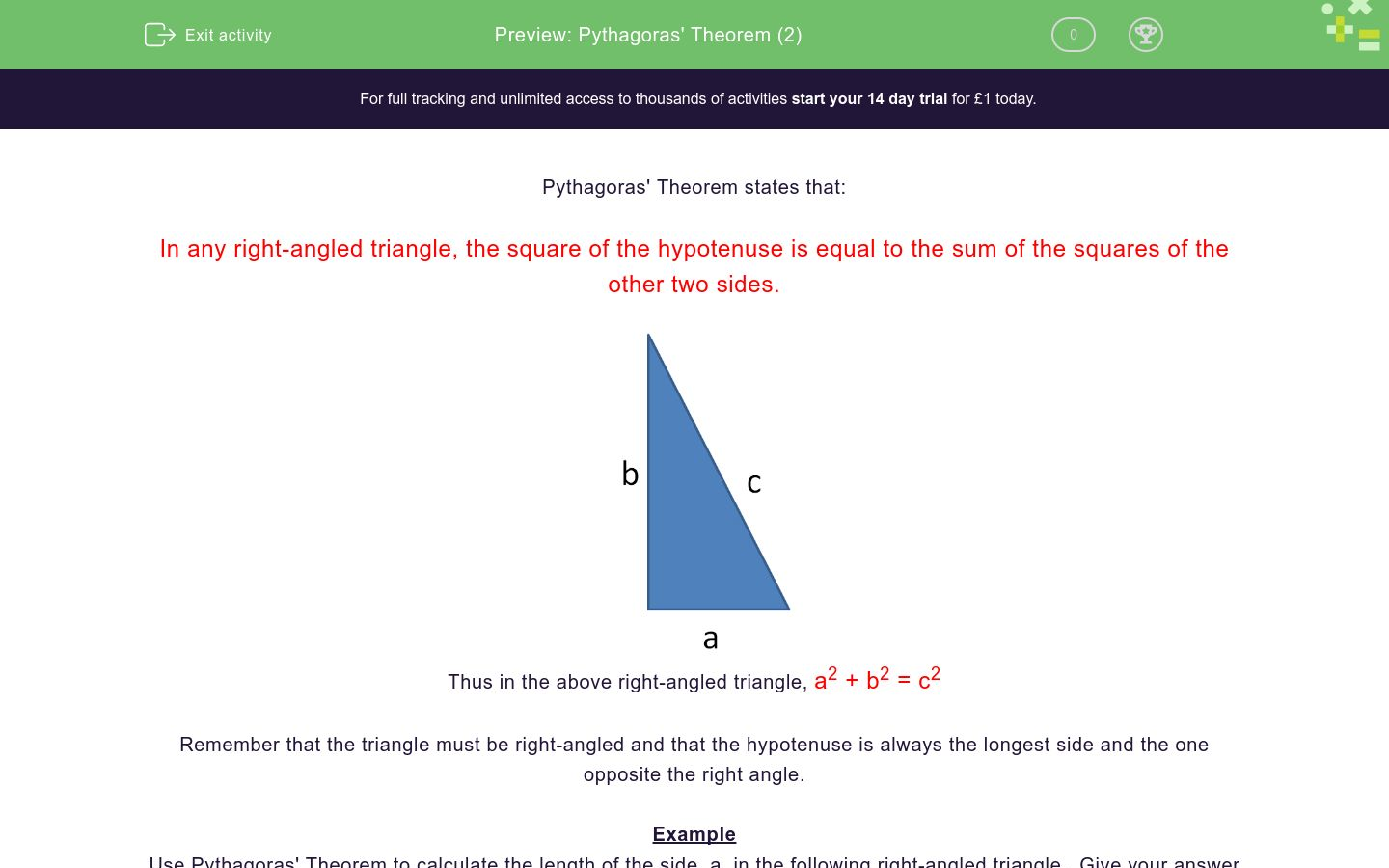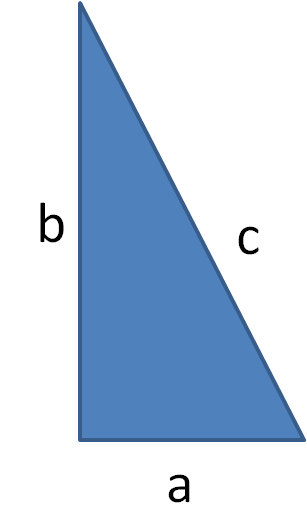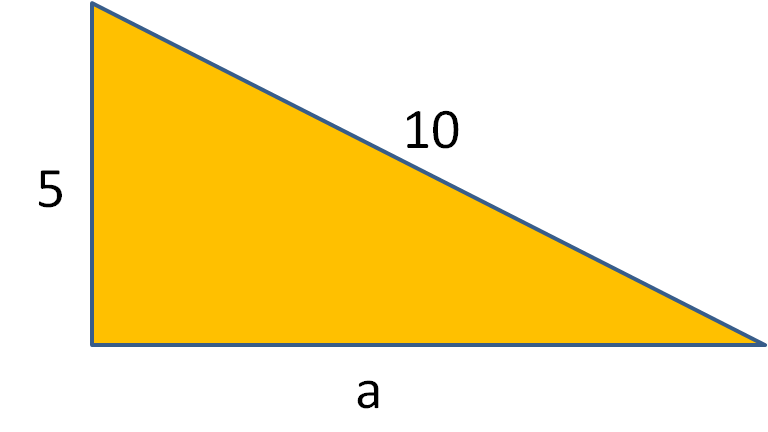# Pythagoras' Theorem (2)

In this worksheet, students find the length of one of the shorter sides of right-angled triangles using Pythagoras' Theorem.Key stage:  KS 3

Curriculum topic:   Geometry and Measures

Curriculum subtopic:   Solve Right-Angled Triangle Problems

Difficulty level:### QUESTION 1 of 10

Pythagoras' Theorem states that:

In any right-angled triangle, the square of the hypotenuse is equal to the sum of the squares of the other two sides.Thus in the above right-angled triangle, a2 + b2 = c2

Remember that the triangle must be right-angled and that the hypotenuse is always the longest side and the one opposite the right angle.

Example

Use Pythagoras' Theorem to calculate the length of the side, a, in the following right-angled triangle.  Give your answer to 3 significant figures.By Pythagoras' Theorem,

a2 + 52 = 92

a2 + 25 = 81

a2 = 81 - 25 =56

a = √56

c = 7.48 (to 3 s.f.)

Use Pythagoras' Theorem to calculate the length of the side, a, in the following right-angled triangle.  Give your answer to 3 significant figures.Use Pythagoras' Theorem to calculate the length of the side, a, in the following right-angled triangle.  Give your answer to 3 significant figures.Use Pythagoras' Theorem to calculate the length of the side, a, in the following right-angled triangle.  Give your answer to 3 significant figures.Use Pythagoras' Theorem to calculate the length of the side, a, in the following right-angled triangle.  Give your answer to 3 significant figures.Use Pythagoras' Theorem to calculate the length of the side, a, in the following right-angled triangle.  Give your answer to 3 significant figures.Use Pythagoras' Theorem to calculate the length of the side, a, in the following right-angled triangle.  Give your answer to 3 significant figures.Use Pythagoras' Theorem to calculate the length of the side, a, in the following right-angled triangle.  Give your answer to 3 significant figures.Use Pythagoras' Theorem to calculate the length of the side, a, in the following right-angled triangle.  Give your answer to 3 significant figures.Use Pythagoras' Theorem to calculate the length of the side, a, in the following right-angled triangle.  Give your answer to 3 significant figures.Use Pythagoras' Theorem to calculate the length of the side, a, in the following right-angled triangle.  Give your answer to 3 significant figures.• Question 1

Use Pythagoras' Theorem to calculate the length of the side, a, in the following right-angled triangle.  Give your answer to 3 significant figures.8.66
EDDIE SAYS

a2 + b2 = c2

a2 + 52 = 102

a2 + 25= 100

a2 = 75

a =√75

• Question 2

Use Pythagoras' Theorem to calculate the length of the side, a, in the following right-angled triangle.  Give your answer to 3 significant figures.9.17
EDDIE SAYS

a2 + b2 = c2

a2 + 42 = 102

a2 + 16= 100

a2 = 84

a =√84

• Question 3

Use Pythagoras' Theorem to calculate the length of the side, a, in the following right-angled triangle.  Give your answer to 3 significant figures.11.3
EDDIE SAYS

a2 + b2 = c2

a2 + 42 = 122

a2 + 16 = 144

a2 = 128

a =√128

• Question 4

Use Pythagoras' Theorem to calculate the length of the side, a, in the following right-angled triangle.  Give your answer to 3 significant figures.6.32
EDDIE SAYS

a2 + b2 = c2

a2 + 32 = 72

a2 + 9= 49

a2 = 40

a =√40

• Question 5

Use Pythagoras' Theorem to calculate the length of the side, a, in the following right-angled triangle.  Give your answer to 3 significant figures.7.42
EDDIE SAYS

a2 + b2 = c2

a2 + 32 = 82

a2 + 9 = 64

a2 = 55

a =√55

• Question 6

Use Pythagoras' Theorem to calculate the length of the side, a, in the following right-angled triangle.  Give your answer to 3 significant figures.5.29
EDDIE SAYS

a2 + b2 = c2

a2 + 62 = 82

a2 + 36 = 64

a2 = 28

a =√28

• Question 7

Use Pythagoras' Theorem to calculate the length of the side, a, in the following right-angled triangle.  Give your answer to 3 significant figures.8.49
EDDIE SAYS

a2 + b2 = c2

a2 + 72 = 112

a2 + 49= 121

a2 = 72

a =√72

• Question 8

Use Pythagoras' Theorem to calculate the length of the side, a, in the following right-angled triangle.  Give your answer to 3 significant figures.5.66
EDDIE SAYS

a2 + b2 = c2

a2 + 72 = 92

a2 + 49= 81

a2 = 32

a =√32

• Question 9

Use Pythagoras' Theorem to calculate the length of the side, a, in the following right-angled triangle.  Give your answer to 3 significant figures.11.6
EDDIE SAYS

a2 + b2 = c2

a2 + 112 = 162

a2 + 121 = 256

a2 = 135

a =√135

• Question 10

Use Pythagoras' Theorem to calculate the length of the side, a, in the following right-angled triangle.  Give your answer to 3 significant figures.10.7
EDDIE SAYS

a2 + b2 = c2

a2 + 92 = 142

a2 + 81= 196

a2 = 115

a =√115

---- OR ----

Sign up for a £1 trial so you can track and measure your child's progress on this activity.

### What is EdPlace?

We're your National Curriculum aligned online education content provider helping each child succeed in English, maths and science from year 1 to GCSE. With an EdPlace account you’ll be able to track and measure progress, helping each child achieve their best. We build confidence and attainment by personalising each child’s learning at a level that suits them.

Get started